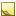﻿ GeometryTools.GetAngleBetweenLines MethodCalculate minimum angle between two lines measured in [0, 90] degrees range.

Namespace:  Accord.Math.Geometry
Assembly:  Accord.Math (in Accord.Math.dll) Version: 3.8.0Syntax
```public static float GetAngleBetweenLines(
Point a1,
Point a2,
Point b1,
Point b2
)```

#### Parameters

a1
Type: AccordPoint
A point on the first line.
a2
Type: AccordPoint
Another point on the first line.
b1
Type: AccordPoint
A point on the second line.
b2
Type: AccordPoint
Another point on the second line.

#### Return Value

Type: Single
Returns minimum angle between two lines.Exceptions
ExceptionCondition
ArgumentExceptiona1 and a2 are the same, -OR- b1 and b2 are the same.RemarksNote
It is preferred to use GetAngleBetweenLines(Line) if it is required to calculate angle multiple times for one of the lines.See Also